# Vegetable meal

The cook was doing meal - in ratio 4: 3: 1 mix tomatoes: pepper: onion. Onions were 5 kg less than peppers. How many kgs of tomatoes did he need to prepare the meal?

r =  10 kg

### Step-by-step explanation: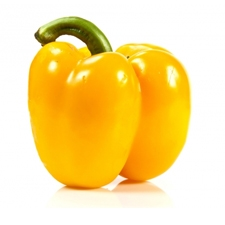Did you find an error or inaccuracy? Feel free to write us. Thank you!Tips to related online calculators
Check out our ratio calculator.
Do you have a linear equation or system of equations and looking for its solution? Or do you have a quadratic equation?

## Related math problems and questions:

• Vegetable stewThe recipe for vegetable stew is recommended to mix tomatoes, peppers, and onions in a ratio of 4: 3: 1. The cook has already prepared both onions and peppers, while the onions were five kg less than peppers. How many kilograms of tomatoes will he need ac
• Shopping vegetablesJohn and his mother were shopping. My mother bought 2 kg of peaches and 5 kg of potatoes and paid CZK 173. The neighbor bought 3 kg of peaches and 4 kg of potatoes and paid CZK 186. How much did 1 kg of peaches and 1 kg of potatoes cost?
• Mixture of coffee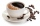They have to prepare a mixture of coffee in packing centres so that 1 kg costs 240 CZK. There are two types of coffee in stock at the price of 220 CZK per 1 kg and 300 CZK per 1 kg. How many kilograms of each species need to be mixed to prepare 50 kg of t
• Vegan shopTo the shops brought together 23.2 kg of goods, fruits, vegetables and nuts. fruit was 4.7 kg more than vegetables, nuts was 1.5 kg less than the fruit. Determine the amount of fruits, vegetables and nuts.
• Crates 2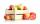In 6 crates is 45 kg of apples In  5 crates is equally In 1 in crate is 3 kg more How many kilograms in each crate?
• Apples 5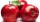In six crates are 45 kg of apples. In five crates were the same amount and in one crate was 3 kg of apples more. How many kg of apples were in each crate?
• SweetsWe want to prepare 5 kg of sweets for 150 CZK. We will mix cheaper candy: 1 kg for 120 CZK and more expensive candy: 1 kg per 240 CZK. How much of these two types of candy is necessary to prepare this mixture?
• MixingIf we mix 5 kg of goods of one kind and 3 kg second one, resulting mixture cost 16.50 EUR/kg. If these quantities are mixed in reverse - first three kilograms and 5 kilograms second cost of mixture is 18.50 EUR/kg. What is the price of one kg of goods of
• Center of gravityThe mass points are distributed in space as follows - specify by coordinates and weight. Find the center of gravity of the mass points system: A1 [1; -20; 3] m1 = 46 kg A2 [-20; 2; 9] m2 = 81 kg A3 [9Grandfather gathered apples into baskets. He filled one large and three small baskets. Small baskets are identical and each can hold 3 kg of apples less than in a large basket. In small baskets together 3 kg of apples over a large basket. How many kilogra
• Bag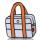Nelly found interesting point. An empty bag weighs 4 kg less than full. And empty bag is 5 times easier than full. How many kg weigh things in this bag?
• Fruit importsCarriers import 18,324 kg of oranges from Spain. It was 16,857 kg less than mandarins and 2,645 kg more than bananas. a) how many kg did they bring mandarins? b) how many kg did bananas bring? c) how much total fruit?
• Barrel 4Barrel of water weighs 63 kg. After off 75% water, the weight of the barrel with water is 21 kg. How many kg weigh empty barrel and how many kgs water in it?
• Six hensSix hens weigh 3 kg more than three geese and 7 kg less than five geese. How much does a hen weigh, and how many geese?
• Potatoes bags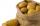I have three bags with 21 kg potatoes. The first bag is 5.5 kg more than in the second bag and the third is 0.5 kg more than in the second bag. Determine how many kgs of potatoes is in each bag.
• Stew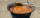To prepare stew for 10 loggers we need: 2.5 kg of potatoes, 0.8 kg of meat, 18 dag of flour, 30 dag onion and 1 bread. What is the required amount of raw materials for 50 participants camps where loggers eats 1.5 times more than a participant of the camp?
• Bent scaleMonica weighed 52 kg. Sara 54 kg. Together they weighed 111 kg. They noticed that the weight on the scale was bent. How much did they really weigh?